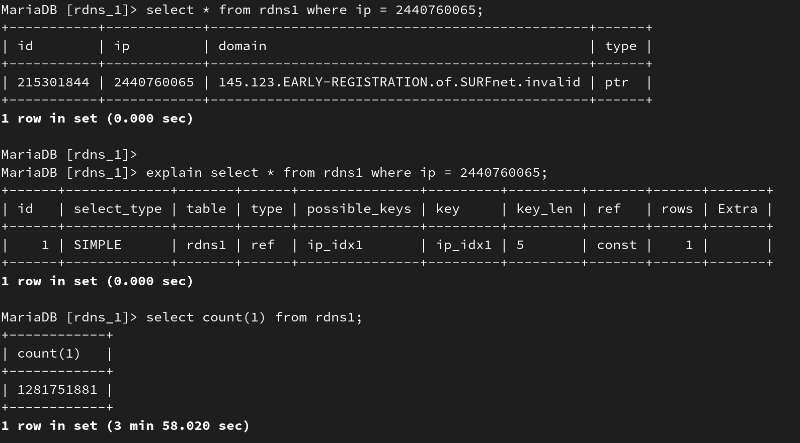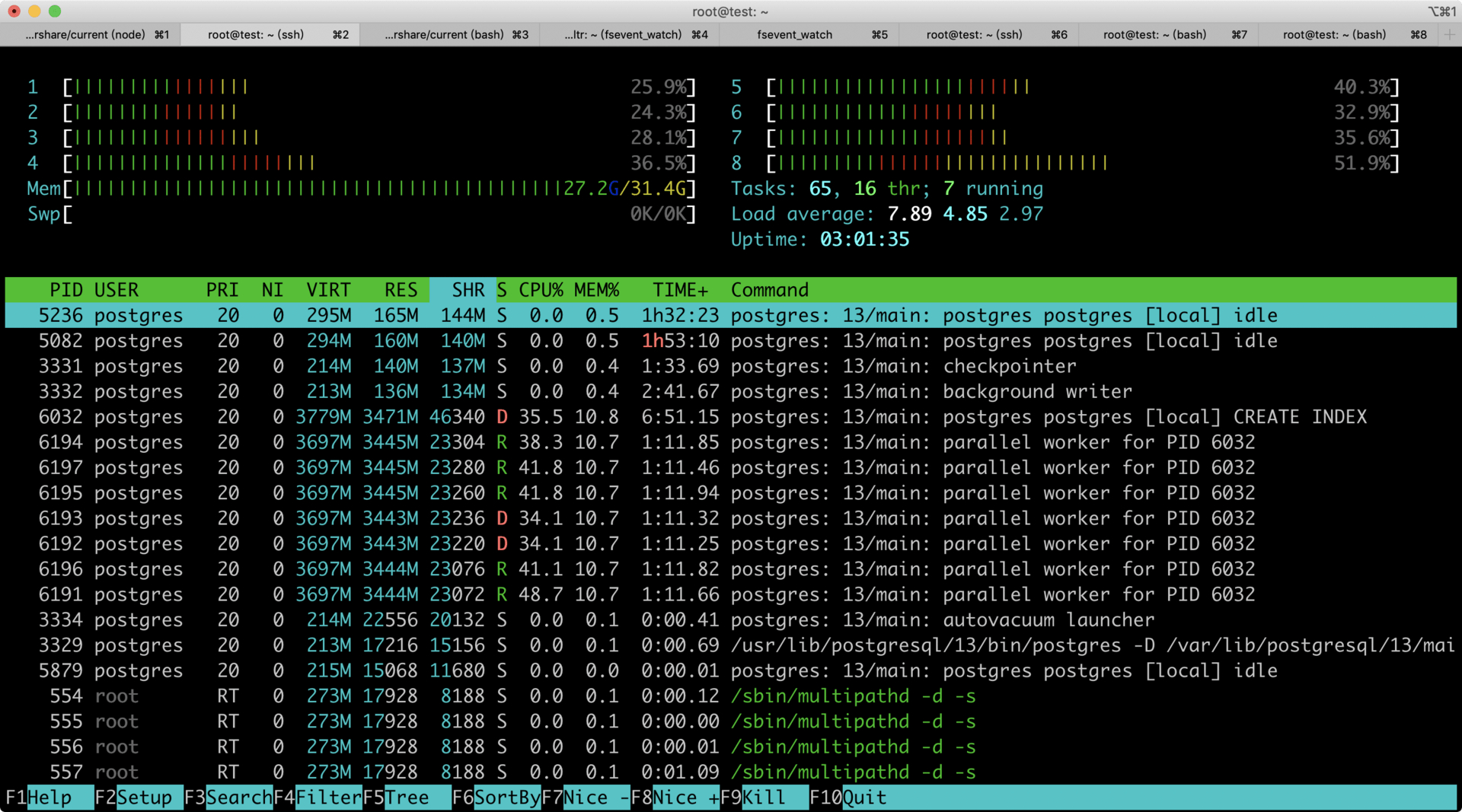# 数据库 单表 13 亿记录创建索引需要多长时间？

hooopo · 2020年10月07日 · 最后由 hooopo 回复于 2020年10月14日 · 3248 次阅读

13 亿 IP 地址，必须要做读写分离了，读库也应该分表了。建议 5000W 一张表。如果 sharding 算法速度快的话，建议 1000W 一张表。1000W 数据停机建立索引，10 分钟应该可以了。你 64C，估算 130 张表 24 小时可以跑完。``````CREATE unlogged TABLE  "test" (
"id" SERIAL PRIMARY KEY NOT NULL,
"ip" integer NOT NULL,
"domain" varchar DEFAULT 'drawerd.com'
);
``````

``````insert into test(ip) select generate_series(1,1280000000);
``````

``````\dt+ test
List of relations
Schema | Name | Type | Owner | Persistence | Size | Description
--------+-------+-------+----------+-------------+-------+-------------
public |  test | table | postgres | unlogged | 62 GB |
``````

``````SET maintenance_work_mem to '30GB';
SET max_parallel_maintenance_workers to 8;
ALTER TABLE test SET (parallel_workers = 8);
``````

``````CREATE INDEX idx ON test (ip);
````````````CREATE unlogged TABLE "ptest" (
"id" SERIAL PRIMARY KEY NOT NULL,
"ip" integer NOT NULL,
"domain" varchar DEFAULT 'drawerd.com'
) partition by hash(id);

create table dept_1 partition of ptest FOR VALUES WITH (MODULUS 8, REMAINDER 0);
create table dept_2 partition of ptest FOR VALUES WITH (MODULUS 8, REMAINDER 1);
create table dept_3 partition of ptest FOR VALUES WITH (MODULUS 8, REMAINDER 2);
create table dept_4 partition of ptest FOR VALUES WITH (MODULUS 8, REMAINDER 3);
create table dept_5 partition of ptest FOR VALUES WITH (MODULUS 8, REMAINDER 4);
create table dept_6 partition of ptest FOR VALUES WITH (MODULUS 8, REMAINDER 5);
create table dept_7 partition of ptest FOR VALUES WITH (MODULUS 8, REMAINDER 6);
create table dept_8 partition of ptest FOR VALUES WITH (MODULUS 8, REMAINDER 7);
``````

"insert into test(ip) select generate_series(1,1280000000);"

``````cat scripts/json2csv.py
import fileinput
import json
import csv
import sys

writer = csv.writer(sys.stdout, quoting=csv.QUOTE_ALL)
for line in fileinput.input():
value = data.get('value')
t = data.get('type')
writer.writerow([ip, value, t])
``````

``````for sf in \$(ls source_file)
do
cat source_file/\$sf | python3 scripts/json2csv.py > target_csv/\$sf.csv &
done
``````

``````mysql> LOAD DATA INFILE '/data/mysql/ii.csv' INTO TABLE `ip` FIELDS TERMINATED BY ',' ;
Query OK, 1280000000 rows affected (1 hour 9 min 55.01 sec)
Records: 1280000000  Deleted: 0  Skipped: 0  Warnings: 0
``````

``````mysql> alter table `ip` add key `idx_ip` (`ip`);
Query OK, 0 rows affected (43 min 54.54 sec)
Records: 0  Duplicates: 0  Warnings: 0
``````

``````70521 be/4 mysql     924.43 K/s  280.63 M/s  0.00 %  0.68 % mysqld --daemonize --pid-file=/run/mysqld/mysqld.pid
``````

• create table 没加 unlogged
• cerate index 的 workers 设置到 32 个。

``````postgres=# CREATE INDEX idx ON test (ip);

Time: 781496.452 ms (13:01.496)
``````

``````at 30s, row rate 666546.92/sec (period), row rate 666546.92/sec (overall), 2.000000E+07 total rows
at 1m0s, row rate 653333.72/sec (period), row rate 659940.91/sec (overall), 3.960000E+07 total rows
at 1m30s, row rate 530000.43/sec (period), row rate 616630.03/sec (overall), 5.550000E+07 total rows
at 2m0s, row rate 546666.11/sec (period), row rate 599139.82/sec (overall), 7.190000E+07 total rows
at 2m30s, row rate 546659.98/sec (period), row rate 588644.12/sec (overall), 8.830000E+07 total rows
at 3m0s, row rate 530004.70/sec (period), row rate 578871.27/sec (overall), 1.042000E+08 total rows
at 3m30s, row rate 543335.38/sec (period), row rate 573794.86/sec (overall), 1.205000E+08 total rows
at 4m0s, row rate 523331.66/sec (period), row rate 567487.09/sec (overall), 1.362000E+08 total rows
``````

4 分钟插入了一亿多条数据，13 亿差不多也得 40 分钟左右。

``````at 30s, row rate 1826650.79/sec (period), row rate 1826650.79/sec (overall), 5.480000E+07 total rows
at 1m0s, row rate 2005766.79/sec (period), row rate 1916228.49/sec (overall), 1.150000E+08 total rows
at 1m30s, row rate 1990897.15/sec (period), row rate 1941106.77/sec (overall), 1.747000E+08 total rows
``````